# Maxima and Minima with Lagrange multipliers (vector calculus)

WMDhamnekar
MHB
Homework Statement:
Find the volume of the largest rectangular parallelpiped that can be inscribed in the ellipsoid
##\frac{x^2}{a^2} + \frac{y^2}{b^2} + \frac{z^2}{c^2} = 1##
Relevant Equations:
No equation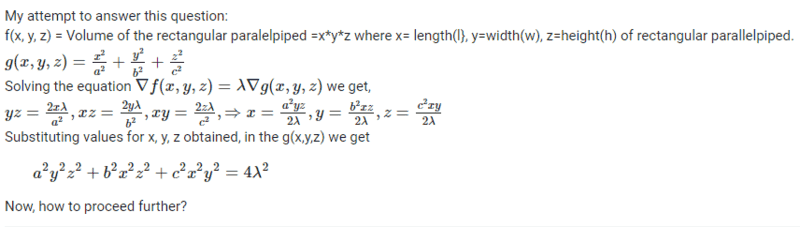Last edited:
•Delta2

Homework Helper
Hi,

Your problem has a multitude of short-cut possibilities. You distract yourself with your substitution.
With three equations in four unknowns you need a fourth. Any suggestions ? (hint: what is it you want to maximize ?)

General advice: if you don't succeed at first, try a simpler case. In this case you could set ##a=b=1## and maximize ##f=xy## subject to ##g = \sqrt{x^2+y^2}\le 1## with a well known answer -- but the point is to understand the method.

##\ ##

WMDhamnekar
MHB
Hi,

Your problem has a multitude of short-cut possibilities. You distract yourself with your substitution.
With three equations in four unknowns you need a fourth. Any suggestions ? (hint: what is it you want to maximize ?)

General advice: if you don't succeed at first, try a simpler case. In this case you could set ##a=b=1## and maximize ##f=xy## subject to ##g = \sqrt{x^2+y^2}\le 1## with a well known answer -- but the point is to understand the method.

##\ ##
Author provided the answer: ## \frac{8abc}{3\sqrt{3}}## I don't understand how was that computed?

Homework Helper
The answer is NOT interesting (but you can check it with ##a=b=c=1## if you insist)

You chose to ignore my advice and my hint. Any particular reason ?
(I could easily give you straight directions to get the answer, but, again, that's not the point of this exercise...)

To clarify:
hint: what is it you want to maximize
use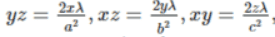to write ##f## as a function of ##x,y,z,\lambda##. Turns out to be very simple...

PS why did you post a picture instead of ##\LaTeX## typesetting ?

##\ ##

WMDhamnekar
MHB
The answer is NOT interesting (but you can check it with ##a=b=c=1## if you insist)

You chose to ignore my advice and my hint. Any particular reason ?
(I could easily give you straight directions to get the answer, but, again, that's not the point of this exercise...)

To clarify:

use View attachment 301199 to write ##f## as a function of ##x,y,z,\lambda##. Turns out to be very simple...

PS why did you post a picture instead of ##\LaTeX## typesetting ?

##\ ##
Maximize f(x,y,z) = volume of rectangular parallelpiped.
If we substitute a=b=c=1 in g(x,y,z), we get equation of sphere ##x^2 + y^2 + z^2 =1 \Rightarrow x=y=z=\frac{\sqrt{3}}{3} f(x,y,z) = \frac{1}{3\sqrt{3}} ## but as per author's answer it must be ##\frac{8abc}{3\sqrt{3}}= \frac{8}{3\sqrt{3}}##

I posted this question on other math help websites also having latex software, to get different ideas of solving the same question from various different math professionals and then analyze them according to its convenience to me. That's why I preferred to post a picture rather than using latex which is time consuming exercise.

Homework Helper
The cube for which was calculated a corner ##x,y,z## has seven other corners. Hence the factor 8.

What did you find and how did you find it ?

##\ ##

•WMDhamnekar
Homework Helper
So what's the status now ? Factor 8 clear ?

##\ ##

WMDhamnekar
MHB
So what's the status now ? Factor 8 clear ?

##\ ##
This question was solved.
## \frac{x^2}{a^2} + \frac{y^2}{b^2} + \frac{z^2}{c^2} = \frac{4xyz}{\lambda}##

and

##\frac{x^2}{a^2} + \frac{y^2}{b^2} + \frac{z^2}{c^2} =1##

##\therefore \frac{x^2}{a^2} + \frac{y^2}{b^2} + \frac{z^2}{c^2} =\frac13##

•BvU
Homework Helper
Strange. With your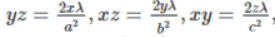I get \begin{align*} xyz & = 2\lambda {x^2\over a^2 }\ ,\\ xyz & =2\lambda{ y^2\over b^2} \ ,\\ xyz & =2\lambda{ z^2\over c^2}\quad \end{align*}so not your
##\quad\ \ \frac{x^2}{a^2} + \frac{y^2}{b^2} + \frac{z^2}{c^2} = \frac{4xyz}{\lambda}##
but ##{x^2\over a^2 }={y^2\over b^2 }={z^2\over c^2 }##
and ##\frac{x^2}{a^2} + \frac{y^2}{b^2} + \frac{z^2}{c^2} = {2\over 3} \frac{xyz}{\lambda}##

?

https://www.whitman.edu/mathematics/calculus_online/section14.08.html
Example 14.8.1 is the example I was referring to (with ##a=b=c=1##).

•WMDhamnekar
Homework Helper
Your
##\qquad\therefore \frac{x^2}{a^2} + \frac{y^2}{b^2} + \frac{z^2}{c^2} =\frac13##
is very far from the constraint !

WMDhamnekar
MHB
Your
##\qquad\therefore \frac{x^2}{a^2} + \frac{y^2}{b^2} + \frac{z^2}{c^2} =\frac13##
is very far from the constraint !
I am very sorry.There are mistakes in the my answer given in #8.

and
##\therefore \frac{x^2}{a^2} = \frac{y^2}{b^2} =\frac{z^2}{c^2}= \frac13##

Last edited:
Homework Helper
Where does the ##1\over 3## come from all of a sudden?

WMDhamnekar
MHB
Where does the ##1\over 3## come from all of a sudden?
Please use some algebra to find out that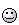Homework Helper
I'm not trying to find out that. I am asking you something

WMDhamnekar
MHB
I'm not trying to find out that. I am asking you something
Hi,
Let P(x, y, z) be a point on the ellipsoid. The 8 vertices of the rectangular parallelpiped are ## P(\pm x, \pm y , \pm z).## Therefore maximize Volume(V) of the rectangular parallelpiped## f(x,y,z)=V = (2x\cdot 2y \cdot 2z)= 8xyz##

given

##g (x,y,z) = \frac{x^2}{a^2} + \frac{y^2}{b^2} + \frac{z^2}{c^2}=1##

Now, read my post #1 and #11, use some algebra you will get the answer ##\frac{8abc}{3\sqrt{3}}## as given by author. I got the answer which correctly matches with the author's answer.
That's it.

•Delta2
Homework Helper
Nobody is interested in the answer. What you want to get out of this exercise is an understanding of the Lagrange multipliers method.

the largest rectangular parallelpiped that can be inscribed in the ellipsoid...

Which is different from
given
$$g (x,y,z) = \frac{x^2}{a^2} + \frac{y^2}{b^2} + \frac{z^2}{c^2}\ \ = \ \ 1$$

At some point (imho) you explicitly need to make the step from ##g\le 1## to ##g=1##. Perhaps you did, but then I missed that.

(see example 4 here)

Other than that, you did fine. Even without following the suggestions in #2 ...

Well, apart also from setting up the wrong ##f## in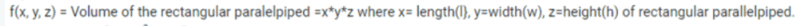##\ ##

•WMDhamnekar
Prof B
Where does the ##1\over 3## come from all of a sudden?
I'm not trying to find out that. I am asking you something
3 equal numbers add up to 1. Are you really questioning how he deduced that each number is 1/3?

Prof B
At some point (imho) you explicitly need to make the step from ##g\le 1## to ##g=1##. Perhaps you did, but then I missed that.
Inscribed means that all corners are on the surface. That's why g=1.

•BvU
Homework Helper
Gold Member
I think post #15 gives the answer, as well correcting the initial mistake in the objective function.

Homework Helper
Gold Member
I have noticed several similar questions over the past year or so about a 2 or 3-variable Lagurange optimisation problem in each of which the answer would have come out immediately if you consider the symmetry of the problem.

A problem symmetric in several variables should have a solution symmetric in those variables.

Unlike the other problems this one requires a slight tweak at the beginning to make it symmetric. Just change the variables into three others: ##X=ax, Y=by, Z=cZ##, then the problem becomes find the volume of the cube whose corners are points on the sphere ##X^2+Y^2 +Z^2=1##, the volume of the parallipiped is then ##abc## times that.

The side of the cube whose corners are on a sphere of radius 1 is 2/√ 3
so giving a cube of volume 8/3√3 and parallelipiped volume 8abc/3√3.

The maxima in the other problems pwere obtainable by making other variables equal to the variable ##x## and finding the ##x## that maximised that singl-variable function, very simple. Of course you need to know Lagrange to be able to deal with the general case, and the right answer was always found - just I thought they were rather here's a problem about a formula, here's a method, turn the handle, here's another formula which is the solution, but the somewhat intriguing geometry of the functions went unnoticed.

•Delta2